# Calculus Homework Help

#### Contact Us

You can e-mail us your problems for a free quote.

SAMPLE CALCULUS PROBLEMS

Problem 1. Find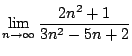Solution.

The problem presents the typical case of a quotient of two quantities that go to infinity. In fact,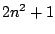and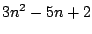both approach to infinity as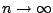. Which means that the quotient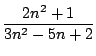is an undetermined form. Somehow we have to cancel the "bad" part, if possible. Notice that since bothandare big, there's a chance for cancelation, so the quotient could be finite.

The trick in this case is to divide by the highest power on the expression (from both numerator and denominator). In this case we will divide both numerator and denominator by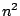. We getasbecause,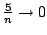and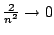as.

This trick is applied in general for this type of expressions.Problem 2. Find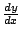and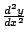if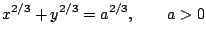Solution. Let's differentiate both sides of the equality. Let's recall that we are assuming that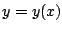. That means that the equation gives us a way to solve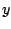as a function of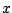, but we still don't know how to express that function. That assumption requires further justification (meaning, it's not always granted), but we'll take it for granted. So, sincewe get using the chain rule:where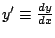. The 0 on the right hand side comes from differentiating the constant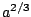. We multiply the equation by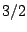and we get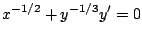which means that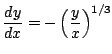Let's get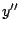. From the last equation we obtain easily that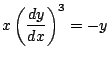and now we differentiate this again with respect toto get:But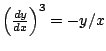and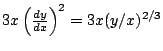. We know put all the elements together:and now we simplify the right hand side of the last equation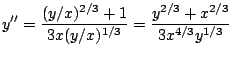But by definition: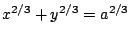, so we replace this into the previous equation:which gives the final answer.